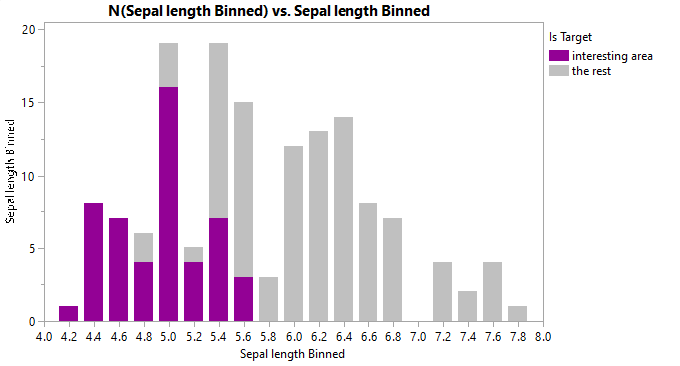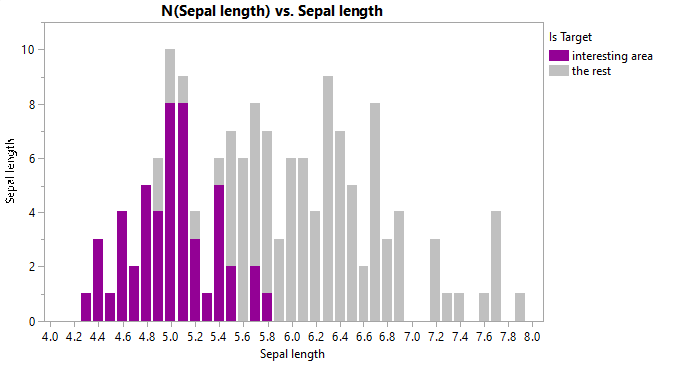Choose Language Hide Translation Bar
Highlighted

## Control bin width in bar chart with

If you drop the sepal length column from the iris data set on both the x and y axes, then choose a bar chart with count as the summary statistic and stacked as the bar style you are presented with a stacked histogram.  Is it possible to change the bin width on the x axis for this chart?

Desired chart:The bar chart using a continuous variable:Adding a bar style chart to the histogram would be a more intuitive method but there is no bar style option there.  I did submit a wish list item to add that here.

Script to make charts:

View more...
``````Names default to here(1);

dt = Open( "\$Sample_Data/iris.jmp" );

dt << New Column("Sepal length Binned", Numeric, "Continuous", Format("Fixed Dec", 12, 1), Formula(Floor(:Sepal length / 0.2) * 0.2));
dt << New Column("Is Target", Character, "Nominal", Set Property("Value Colors", {"Is Target" = 59, "The Rest" = 32}), Formula(If(:Species == "setosa", "Is Target", "The Rest")));

//Desired Chart - requires extra column
dt << Graph Builder(
Size( 500, 300 ),
Show Control Panel( 0 ),
Variables(
X( :Sepal length ),
Y( :Sepal length ),
Overlay( :Is Target ),
Color( :Is Target )
),
Elements(
Bar( X, Y, Legend( 11 ), Bar Style( "Stacked" ), Summary Statistic( "N" ) )
),
SendToReport(
Dispatch(
{},
"Sepal length",
ScaleBox,
{Min( 4.1659880239521 ), Max( 8.0340119760479 ), Inc( 0.2 ),
Minor Ticks( 0 )}
)
)
);

//Automatic Bin Width - easier
dt << Graph Builder(
Size( 500, 300 ),
Show Control Panel( 0 ),
Variables(
X( :Sepal length Binned ),
Y( :Sepal length Binned ),
Overlay( :Is Target ),
Color( :Is Target )
),
Elements(
Bar( X, Y, Legend( 11 ), Bar Style( "Stacked" ), Summary Statistic( "N" ) )
),
SendToReport(
Dispatch(
{},
"Sepal length",
ScaleBox,
{Min( 4.1659880239521 ), Max( 8.0340119760479 ), Inc( 0.2 ),
Minor Ticks( 0 )}
)
)
);

//Histogram option - no way to stack bars, leads to confusion when 'Is Target'
//and 'The rest' have similar counts
dt << Graph Builder(
Size( 500, 300 ),
Show Control Panel( 0 ),
Variables(
X( :Sepal length ),
Y( :Sepal length ),
Overlay( :Is Target ),
Color( :Is Target )
),
Elements( Histogram( X, Y, Legend( 12 ) ) ),
SendToReport(
Dispatch(
{},
"Sepal length",
ScaleBox,
{Min( 4.1659880239521 ), Max( 8.0340119760479 ), Inc( 0.2 ),
Minor Ticks( 0 )}
)
)
);``````
Highlighted

## Re: Control bin width in bar chart with

In this case, using Sepal Length as the X-axis variable, for a bar chart.

Sepal Length is. an continuous numeric variable; however, a bar chart only work with nominal (categorical) variables.

Since sepal length is rounded to the nearest 10th, it is plotted as the number of times each sepal length (in increments of tenths) occurs in the data set.

For example, write this formula in a column

``:Sepal length + Random Normal()``

Use that column in a graph builder plot, and choose bar chart. The result is a haze of lines, or very thin bars.

If you want fewer bins, in this case, rounding the data appropriately could help.

So the short answer is, no you can't change it in the graph. The answer is also, don't make bar charts using a continuous variable for categories. Check out the add-in, Interactive Binning v2.  This tool lets you break data down into bins either manually of with a couple of other statistical intervals.

JMP Systems Engineer, Pharm and BioPharm Sciences
Article Labels

There are no labels assigned to this post.